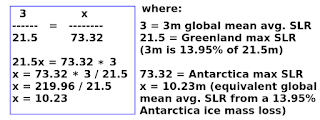## Monday, January 25, 2016

### The Ghost-Water ConstantFig. 1 USGS sea level rise potential modified
In a previous post of a Dredd Blog series, I did some calculations concerning the sea level change (SLC) impact of ice sheet gravity (The Gravity of Sea Level Change - 4).

The result was an additional 11.2m of sea level rise (SLR) for the two main locations considered (Antarctica & Greenland).

The description of the phenomenon was "ghost-water" because an additional amount of SLR will take place without any additional calving or melting of ice sheets.

It is totally the result of losing something that can't be seen: ghostly gravity.

The ghost-water is water initially pulled up against the land mass (Greenland) all along the coastline, by the power of the Greenland ice sheet's gravity.

That ghost-water is released to flow elsewhere as the "ghost-gravity" is lost incrementally when the ice sheet mass decreases due to either ice melt or glaciers calving (The Gravity of Sea Level Change).

The gravity is redistributed along with the melt water, along with the calving ice bergs, and along with the ghost-water.

II. Let's Revisit the Formula

As it turns out, this ghost-water thingy is a constant which I am naming "gW".

I am going to apply it to all ice (Fig. 1) today, and determine its full value.

The numbers seem scary, so I want to double check the figures that Dr. Mitrovica gave us in the video (see video below).

So, we will start from scratch, initially leaving out Antarctica:
V = (b * h * l) / 2 (the formula)

b = 2000 km
h = 20 m
l = 44,087 km (Greenland coastline length)
...
V = (2,000,000 m * 20 m * 44,087,000 m) / 2
V = (1.76348 × 1015 m3) / 2 (meters)
V = (1.76348 × 1012 km3) / 2 (kilometers)
...
(1.76348 × 1012 km3 × 0.00000000278 mm = 4902.4744 mm) / 2
((4902.4744 mm = 16.08 ft. (divide mm by 304.8 to derive feet)) / 2
8.042 feet (2.451m) of additional SLR (by Greenland ghost-water)
(see formula description @ The Gravity of Sea Level Change - 4). Ok, so now let's do Antarctica by itself:
V = (b * h * l) / 2 (the formula)

b = 2000 km
h = 20 m
l = 17,968 km (Antarctica coastline length)
...
V = (2,000,000 m * 20 m * 17,968,000 m) / 2
V = (7.1872 × 1014 m3) / 2 (meters)
V = (3.5936 × 1011 km3) / 2 (kilometers)
...
(3.5936 × 1011 km3 × 0.00000000278 mm = 999.0208 mm) / 2
((999.0208 mm = 3.278 ft. (divide mm by 304.8 to derive feet)) / 2
1.639 feet (0.5m) of additional SLR (by Antarctica ghost-water)
(see  The Gravity of Sea Level Change - 4 ... notice that Greenland and Antarctica were combined in that post). The total is 1.639 ft.+ 8.042 ft. = 9.681 ft. this way, instead of 11.32 the other way.

This, I think, is better than equating Antarctica with Greenland, because they really are not equal (Fig. 2).

Antarctica has less coastline according to the source I used in that previous post.

But Antarctica has more SLC potential, 64.80m + 8.06m + .46m = 73.32m, compared to Greenland's 6.55m (as shown in Fig. 1).

Thus, in the long run, an equal percentage of loss from each ice sheet will result in a larger number for the ghost-water phenomenon.

The lesson is that the circumference, i.e. the length of the coastline (e.g. jagged vs. smooth) has an effect on the outcome of the calculation, as does an ice sheet's total mass.

After Greenland is completely exhausted of its ice mass, Antarctica will still generate regular SLR as well as ghost-water SLR.

Anyway, according to Fig. 1, having done Greenland and Antarctica, that leaves only 0.45m more SLR to consider.

In other words, the non-ice sheet ice reservoirs - e.g. Glacier Bay - have mainly local impact.

III. What is the Constant?

The ghost-water is quite real, and should always be considered in SLC / SLR scenarios, it would seem.Fig. 2 Comparison: Greenland / Antarctica

To make the ghost-water reality more useful, we must link it to the full potential SLR of 80.32m (Fig. 1).

Nevertheless, there may not be an overarching constant at work here.

I mean a mathematical constant like G, the gravitational constant, and the thingy that the Masons put on their rings and stuff.

The exercise Mitrovica did with Greenland was 20m of sea level fall (SLF) around its coasts generating a global mean average SLR of 3m.

The arithmetic in Fig. 2 shows that using the proper ratio will result in an increase of ghost-water SLR.

If the 13.95% holds up, then 13.95% of 80.32 is 11.2 m of additional SLR should also be considered (80.32m + 11.2m = 91.5m [300 ft.] total potential SLR)?

Doesn't this mean that any ice sheet generated SLR contains a significant amount of ghost-water SLR?

That amount of ghost-water would be 13.95% (other factors, such as thermal expansion are minor by comparison).

IV. Conclusion

I am going to keep thinking about this, because there is at least a ratio of ice mass loss to ghost-water action on SLR in other parts of the globe where ghost-water ends up.

So far, the only place it does not end up is in the declarations of those who talk about "king tide" "storm surge" "unusual wa wa in my yard" and "God-water!! says Inhofe" ... "you can't tell one Atlantis from another by just one weather event."

We shall see the gravity of all of this.

The next post in this series is here.

Professor Jerry X. Mitrovica on the gravity / axis bulge SLR / SLF issues we don't hear about often enough:

#### 1 comment:

1.Meanwhile, way too many science professionals are clueless (link).